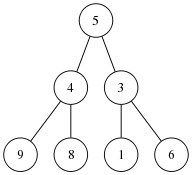## A Simple Application of Continuations

Beginning Scheme programmers—and, indeed, even those with more experience—often have difficulty with continuations. It can be hard to get your head around the concept of continuations. Because they are so powerful and flexible, it sometimes seems as if every use must involve some heavy duty technique.

Here is a simple example that shows how to perform a return from deep within a series of recursive calls. Given a binary tree, such as that below, we want to write a function that will return the path from the root to any specified node in the tree. If the node is not found, the function should return `#f`.Before we begin, we should agree on our representation for the tree. We will use the representation where each node is represented as

```(value (left-subtree) (right-subtree))
```

Thus our sample tree is represented as

```(define tr '(5 (4 (9 () ()) (8 () ())) (3 (1 () ()) (6 () ()))))
```

We will use the standard depth first search technique of recursing down the left subtree and then the right subtree looking for the desired node. When we find it, we would like to return the result immediately. We could do this by having our search routine pass back the path or #f at each call and then act accordingly, but the logic of the function then becomes more complicated. Instead we use `call/cc` to just return immediately.

``` 1:  ;; Return the path from the root of tree to the specified vertex
2:  ;; Each vertex has the form (value (left-substree) (right-subtree))
3:  (define dfs
4:    (lambda (tree vertex)
5:      (letrec ((dfs1 (lambda (tree path ret)
6:                       (unless (null? tree)
7:                         (let* ((value (car tree))
8:                                (new-path (cons value path)))
9:                           (if (= value vertex)
10:                               (ret (reverse new-path)))
11:                           (dfs1 (cadr tree) new-path ret)
12:                           (dfs1 (caddr tree) new-path ret))))))
13:        (call/cc
14:         (lambda (ret)
15:           (dfs1 tree '() ret)
16:           #f)                   ; lambda returns here
17:         )                       ; call/cc returns here
18:        )))
```

All the work is done in the `dfs1 letrec`. We either find the correct node and call the `ret` function (actually, `ret` is a continuation) to return the path (lines 9–10) or we recurse down the left and right subtrees (lines 11–12).

The continuation is created by the `call/cc` on line 13. It takes a single argument, which must be a function of a single variable. In our case, the function is the `lambda` function on lines 14–16. The `call/cc` passes the continuation to its function argument as the function's single argument. If the node isn't in the tree, `dfs1` will return normally and the `call/cc` will return `#f`. If the node is found, calling the continuation causes control to pass to the end of the `call/cc` at line 17. I've abused the formatting a little so that the extent of the `lambda` function and the `call/cc` are clearer.

This example barely hints at the power of continuations but it does show that they can be used to solve everyday problems in an easy and natural way.

Update: Fixed typos and import of picture